# Rhombic triacontahedron

Rhombic triacontahedron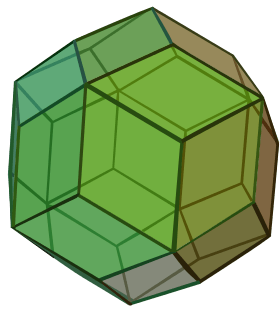TypeCatalan solid
Coxeter diagramConway notationjD
Face typeV3.5.3.5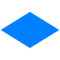rhombus
Faces30
Edges60
Vertices32
Vertices by type20{3}+12{5}
Symmetry groupIh, H3, [5,3], (*532)
Rotation groupI, [5,3]+, (532)
Dihedral angle144°
Propertiesconvex, face-transitive isohedral, isotoxal, zonohedron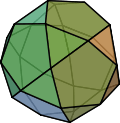Icosidodecahedron
(dual polyhedron)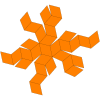Net

In geometry, the rhombic triacontahedron, sometimes simply called the triacontahedron as it is the most common thirty-faced polyhedron, is a convex polyhedron with 30 rhombic faces. It has 60 edges and 32 vertices of two types. It is a Catalan solid, and the dual polyhedron of the icosidodecahedron. It is a zonohedron.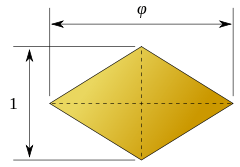A face of the rhombic triacontahedron. The lengths of the diagonals are in the golden ratio.

The ratio of the long diagonal to the short diagonal of each face is exactly equal to the golden ratio, φ, so that the acute angles on each face measure 2 tan−1(1/φ) = tan−1(2), or approximately 63.43°. A rhombus so obtained is called a golden rhombus.

Being the dual of an Archimedean solid, the rhombic triacontahedron is face-transitive, meaning the symmetry group of the solid acts transitively on the set of faces. This means that for any two faces, A and B, there is a rotation or reflection of the solid that leaves it occupying the same region of space while moving face A to face B.

The rhombic triacontahedron is somewhat special in being one of the nine edge-transitive convex polyhedra, the others being the five Platonic solids, the cuboctahedron, the icosidodecahedron, and the rhombic dodecahedron.

The rhombic triacontahedron is also interesting in that its vertices include the arrangement of four Platonic solids. It contains ten tetrahedra, five cubes, an icosahedron and a dodecahedron. The centers of the faces contain five octahedra.

## Dimensions

If the edge length of a rhombic triacontahedron is a, surface area, volume, the radius of an inscribed sphere (tangent to each of the rhombic triacontahedron's faces) and midradius, which touches the middle of each edge are:where φ is the golden ratio.

The plane of each face is perpendicular to the center of the rhombic triacontahedron, and is located at the same distance (short diagonals belong only to the edges of the inscribed regular dodecahedron, while long diagonals are included only in edges of the inscribed icosahedron). Using one of the three orthogonal golden rectangles drawn into the inscribed icosahedron we can easily deduce the distance between the center of the solid and the center of its rhombic face.

## Dissection

The rhombic triacontahedron can be dissected into 20 golden rhombohedra, 10 acute ones and 10 flat ones.

## Uses of rhombic triacontahedra

Danish designer Holger Strøm used the rhombic triacontahedron as a basis for the design of his buildable lamp IQ-light. (IQ for "Interlocking Quadrilaterals")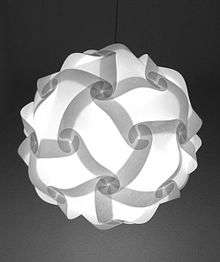An example of the use of a rhombic triacontahedron in the design of a lamp. IQ stands for “Interlocking Quadrilaterals”.

Woodworker Jane Kostick builds boxes in the shape of a rhombic triacontahedron. The simple construction is based on the less than obvious relationship between the rhombic triacontahedron and the cube.

Roger von Oech's "Ball of Whacks" comes in the shape of a rhombic triacontahedron.

In some roleplaying games, and for elementary school uses, the rhombic triacontahedron is used as the "d30" thirty-sided die.

## Orthogonal projections

The rhombic triacontahedron has three symmetry positions, two centered on vertices, and one mid-edge. (embedded in projection "10" are the "fat" rhombus and "skinny" rhombus which tile together to produce the non-periodic tessellation often referred to as "Penrose Tiing")

Projectivesymmetry Image Dualimage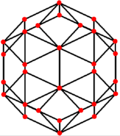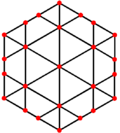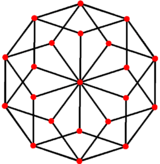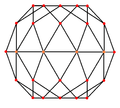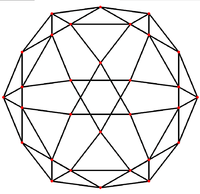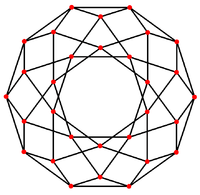### Stellations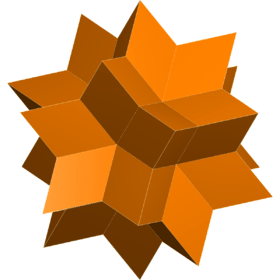Rhombic hexecontahedron
Further information: Rhombic hexecontahedron

The rhombic triacontahedron has over 227 stellations.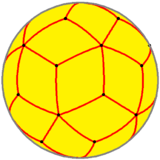Spherical rhombic triacontahedron

This polyhedron is a part of a sequence of rhombic polyhedra and tilings with [n,3] Coxeter group symmetry. The cube can be seen as a rhombic hexahedron where the rhombi are also rectangles.

The rhombic triacontahedron forms the convex hull of one projection of a 6-cube to 3 dimensions.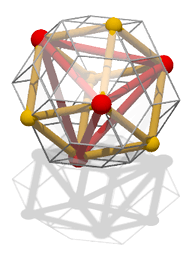A rhombic triacontahedron with an inscribed tetrahedron (red) and cube (yellow).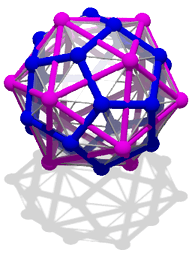A rhombic triacontahedron with an inscribed dodecahedron (blue) and icosahedron (purple).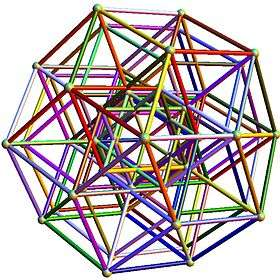The 3D basis vectors [u,v,w] are: u = (1, φ, 0, -1, φ, 0) v = (φ, 0, 1, φ, 0, -1) w = (0, 1, φ, 0, -1, φ)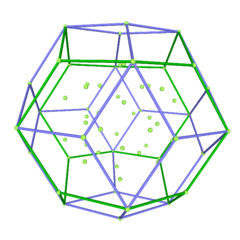Shown with inner edges hidden There are 64 vertices and 192 unit length edges forming pentagonal symmetry along specific axis (as well as hexagonal symmetries on other axis).